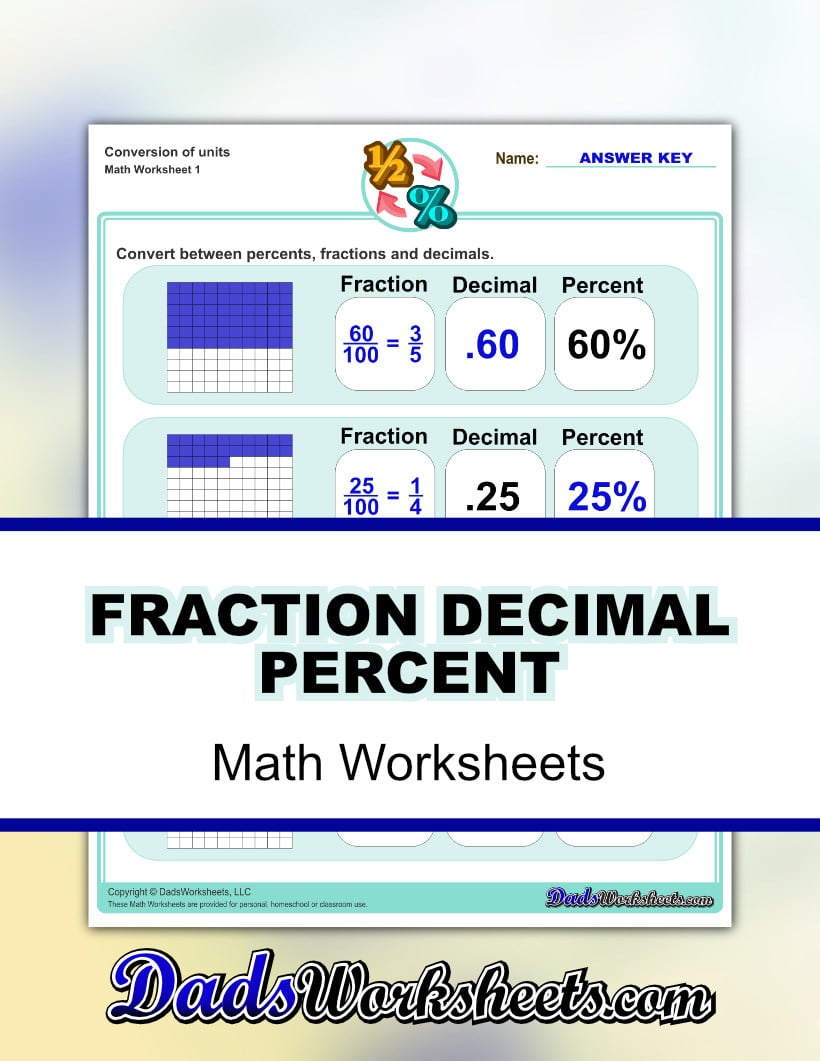# Fraction Decimal Percent Worksheets

The concept that a fraction, a decimal amount and a percentage can all represent the same quantity is very important to solving many real world math problems. Fraction decimal percent worksheets give students practice converting between these three forms and can be a good introduction to more complex concepts in percentage worksheets or certain types of word problems. These worksheets start with more familiar fractional quantities and then gradually move on to more common fractions and percents that every student should be familiar with. The harder sets of worksheets include fractions and percents that are less common, but still great conversion practice.

If you are looking for an amazing tool for visualizing different fractions and their decimal equivalents, be sure to check out this fraction chart which shows families of equivalent fractions in an elegant way along with where they fall on a number line.

## Need More Decimal, Fraction and Percentage Worksheets?

After mastering these fraction decimal percent worksheets, are you looking for more practice with fractional quantities? Check out these fraction resources for more tools appropriate for your 4th or 5th grade students:

## Check Out These Equivalent Fraction Charts!

These fraction charts show where specific groups of equivalent fractions land on the number line with their decimal equivalents. Ready to print for free as a student notebook fraction anchor chart, or order it as a beautiful classroom poster!

Fraction Chart